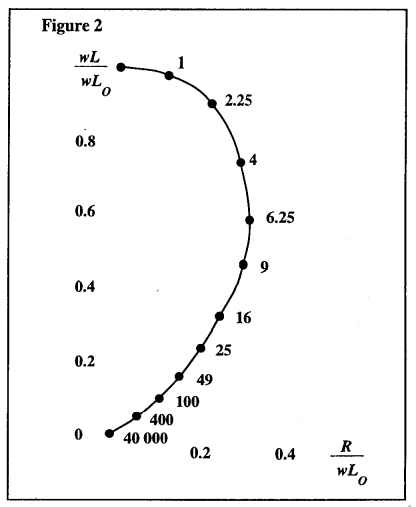# Take A Free ASNT NDT ET Level 2 Exam Practice Test – Quiz Course

Here you can take a free ASNT NDT ET Level 2 Exam Practice Test with the latest Eddy Current Testing Method Level II Questions and Answers.

1.

2.

3.

4.

5.

6.

7.

8.

9.

10.

11.

12.

13.

14.

15.

16.

17.

18.

19.

20.

21.

22.

23.

24.

25.

26.

27.

28.

29.

30.

31.

## The abscissa values on the impedance plane are shown in Figure 2 is given in terms of:32.

## In Figure 2 (an impedance diagram for a solid non-magnetic rod), the fg or characteristic frequency is calculated by the formula:33.

34.

35.

36.

37.

38.

39.

40.

41.

42.

43.

44.

45.

46.

47.

48.

49.

50.

51.

52.

## The formula used to calculate the impedance of an eddy current test coil is:53.

54.

55.

56.

57.

58.

59.

60.

61.

62.

63.

64.

65.

66.

67.

68.

69.

70.

71.

72.

73.

74.

75.

76.

77.

78.

79.

80.

81.

82.

83.

84.

85.

86.

87.

88.

## In Figure 8, the distance represented by C is a measure of the materials:89.

90.

91.

92.

93.

94.

95.

96.

97.

98.

99.

100.

101.

102.

103.

104.

## In modulation analysis testing, which of the following would not modulate the test frequency applied to the test coil?

Question 1 of 104

## Are You Looking for a Free ASNT NDT ET Level 2Exam Practice Test?

If your answer is yes then you are at the right website. Here You Can Take a Free Online ASNT NDT ET Level 2 Exam Practice Test and Improve Your ASNT ET Level II – Electromagnetic Testing Method Exam Score. Here You Can Learn everything you need to know about ASNT ET Level II quickly and easily.

In This Practice test, We are covering the Latest ASNT ET Level 2 – Electromagnetic Testing Method Question and Answers, After completing the last Question you will see the ASNT ET Level II Practice Test Answer Key, No cheating now! Once you complete the test and check your answers please leave us your score and any comments in the comments section below

We will help you to pass the ASNT ET Level 2 Exam on the first attempt —99% Guaranteed. Our expert-written ASNT ET Level II – Electromagnetic Testing Method Examination practice material covers the actual exam topics with fully explained answers.

Take unlimited free ASNT ET Level 2 Quiz Course tests of any length. We have free Latest questions updated every week and you can study our material along with any other study materials.

In this quiz course, we are covering all the topics listed below:

• Principles of Electromagnetic Testing
• Test Coil Arrangements
• Test Coil Design
• Effects of Test Objects on Test Coils
• Selection of Test Frequency
• Instrument Systems
• Eddy Current Applications
• Alternating Current Field Measurement
• Remote Field Testing
• Electromagnetic Testing Procedures, Specifications, and Standards

Buy ASNT ET LEVEL 3 Exam Learning Package Study Material
FOR EVERY 10 QUESTIONS 1 QUESTION COMES INTO THE EXAM
WE HAVE LATEST ASNT ET Level III – Electromagnetic Testing Method QUESTION BANK
Total 2000+ nos QUESTION BANK FROM THEORY TO PRACTICAL TO NUMERICALS.
Cost: \$25.00